• 使用C语言解决八皇后问题。使用回溯法。方便学习，了解回溯法。
• ## c语言八皇后问题

千次阅读 2016-12-05 03:06:30
八皇后问题就是在8*8的棋盘上的每一行都放置一个皇后，使他们无法进行互相攻击 思路： 1.编写一个函数，将一个皇后放在第一列，如果存在攻击就把皇后放在第二列，如果每列都存在互相攻击的情况，则这个函数返回 2....
在国际象棋里面，皇后是最具有杀伤力的武器，它可以对它的十字形和对角线元素进行攻击。
八皇后问题就是在8*8的棋盘上的每一行都放置一个皇后，使他们无法进行互相攻击

思路：
1.编写一个函数，将一个皇后放在第一列，如果存在攻击就把皇后放在第二列，如果每列都存在互相攻击的情况，则这个函数返回
2.若皇后可以放在这个位置，则应该递归的调用本身，把皇后放在下一行，当递归函数返回时候，就证明本行这个位置放置导致下一行皇后无处可放，则将本行皇后向后移动一个位置，判断是否可以放置皇后，如果可以则进入递归函数里面，若不可以则在将本行皇后向后挪动一列
首先我们应该编写检查函数：

int isSafe(int (*chess)[EIGHT], int row, int col)
{
int rowIndex;
int colIndex;

for(rowIndex = row-1; rowIndex >= 0; rowIndex--){//直上方
if(chess[rowIndex][col])
return FALSE;void eightQueen(int (*chess)[EIGHT], int row)
{

int colIndex;

if(row >= EIGHT){
showChess(chess);
}
else{
for(colIndex = 0; colIndex < EIGHT; colIndex++){
//chess[row][colIndex] = 1;
if(isSafe(chess, row, colIndex) == TRUE){//如果可以放置皇后
chess[row][colIndex] = 1;//放置皇后
eightQueen(chess, row+1);//进行递归
chess[row][colIndex] = 0;//递归返回时，证明此列不适合,下一列放置皇后
}
}
}
}

递归函数：void eightQueen(int (*chess)[EIGHT], int row){

int colIndex;

if(row >= EIGHT){
showChess(chess);
}
else{
for(colIndex = 0; colIndex < EIGHT; colIndex++){
//chess[row][colIndex] = 1;
if(isSafe(chess, row, colIndex) == TRUE){//如果可以放置皇后
chess[row][colIndex] = 1;//放置皇后
eightQueen(chess, row+1);//进行递归
chess[row][colIndex] = 0;//递归返回时，证明此列不适合,下一列放置皇后
}
}
}

输出函数：

void showChess(int (*chess)[EIGHT]){
int i;
int j;
static int count;

printf("解%d:\n", ++count);
for(i = 0; i < EIGHT; i++){
for(j = 0; j < EIGHT; j++){
printf("%4d", chess[i][j]);
}
printf("\n");
}
}
主函数：
void main()
{
int chess[EIGHT][EIGHT] = {0};//定义并且初始化棋盘

eightQueen(chess, 0);//进行处理

}


展开全文• 主要介绍了C语言八皇后问题解决方法,简单描述了八皇后问题并结合实例形式分析了C语言基于暴力法与回溯法解决八皇后的具体操作技巧,需要的朋友可以参考下
• C语言求解出八皇后所有解，稍作修改可以推至n皇后问题
• C语言八皇后问题 棋盘是8*8的，所以定义一个8的宏，方便后面的使用，如果以后更改棋盘大小，也只需要更改宏定义就好了，很方便。代码如下： #define WIDTH 8 定义一个8*8（棋盘大小为8），值全为0的二维数组，，...
C语言八皇后问题
棋盘是8*8的，所以定义一个8的宏，方便后面的使用，如果以后更改棋盘大小，也只需要更改宏定义就好了，很方便。代码如下：
#define		WIDTH		8

定义一个8*8（棋盘大小为8），值全为0的二维数组，，后面每放一个皇后，其二维数组上的值对应改变为1（0表示无皇后，1表示有皇后）。代码如下：
chessBoard[WIDTH][WIDTH] = {0};

放置皇后的原则为：每一行放置一个皇后，放置皇后的横行、竖行、45°斜行和135°斜行，都不能放置皇后了。所以你放置每一个皇后的时候，需要考虑这个皇后的竖行、45°行和135°行（肯定是一行放一个，所以横行不用考虑，肯定也是由第一行、第二行、第三行依次往后放，所以后面肯定也没有，也就不用考虑了）。判断棋盘此处是否能放置皇后的代码如下：
boolean isSafe(boolean (*chessBoard)[WIDTH], int row, int col) {
int i;
int j;
//检查左斜上方是否有皇后
for (i = row - 1, j = col - 1; i >= 0 && j >= 0; j--, i--) {
if (chessBoard[i][j] == 1) {
return FALSE;
}
}
//检查上方是否有皇后
for (i = row - 1, j = col; i >= 0; i--) {
if (chessBoard[i][j] == 1) {
return FALSE;
}
}
//检查右斜上方是否有皇后
for (i = row - 1, j = col + 1; i >= 0 && j < WIDTH; i--, j++) {
if (chessBoard[i][j] == 1) {
return FALSE;
}
}

return TRUE;
}

如果判断的位置可以放置皇后，则，返回TRUE。不能则返回FALSE。
下面我们编写一个函数，用来把八皇后进行遍历输出。八皇后位置为1。不是八皇后则位置为0；
void showChess(boolean (*chessBoard)[WIDTH]) {
int row;
int col;
static int no = 0;//静态存储类变量只执行一次，而且延长了局部变量的生命周期，直到程序运行结束以后才释放。

printf("第%d个解:\n", ++no);
for (row = 0; row < WIDTH; row++) {
for (col = 0; col < WIDTH; col++) {
printf("%5d", chessBoard[row][col]);//遍历输出八皇后
}
printf("\n");
}
}

下面就是主要的函数，用递归的方法，让八行都有皇后。对于每一行，需要依次尝试每一个位置，判断其安全性（调用上面的isSafe函数），若安全，则尝试下一行（递归）。那么何时能知到八行都有皇后了呢？当能进行到第九行（二维数组下标为8的时候），就得知其摆出了成功的八皇后，即：row >= WIDTH。然后使用前面写过的函数shouChess()，遍历输出八皇后
void chess(boolean (*chessBoard)[WIDTH], int row) {
int col;

if (row >= WIDTH) {
showChess(chessBoard);
return;
}

for (col = 0; col < WIDTH; col++) {
if (isSafe(chessBoard, row, col)) {
chessBoard[row][col] = TRUE;
chess(chessBoard, row + 1);
chessBoard[row][col] = FALSE;
}
}
}

当你递归到下一行的时候，如果八个位置都不满足，那么执行代码：chessBoard[row][col] = FALSE;
即上一行为1的皇后变为0，横行col++。之后进行判断，下一个位置是否安全。
代码输出一共有92种八皇后，但其具有对称性，所以要除于4，即92/4 = 23种八皇后。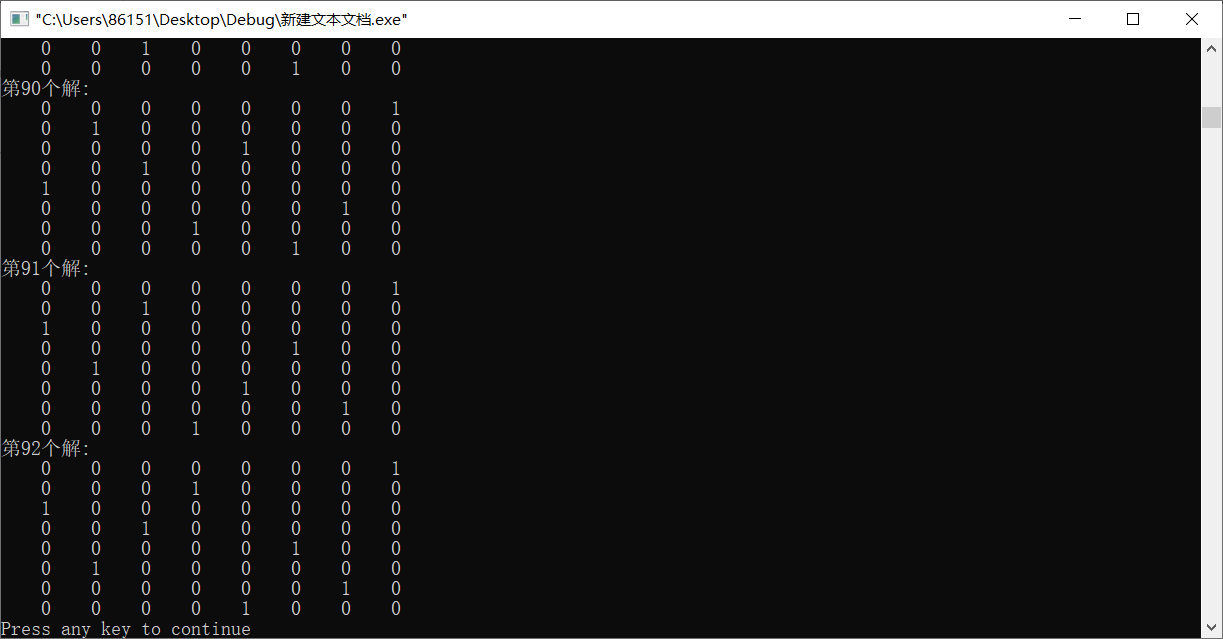以上就是全部的八皇后代码，使用了递归的思想。
后面附上所有的代码（若被使用，请注明出处，谢谢配合）：
#include <stdio.h>

typedef unsigned char boolean;
#define		TRUE		1
#define		FALSE		0

#define		WIDTH		8

void showChess(boolean (*chessBoard)[WIDTH]);
boolean isSafe(boolean (*chessBoard)[WIDTH], int row, int col);
void chess(boolean (*chessBoard)[WIDTH], int row);

void chess(boolean (*chessBoard)[WIDTH], int row) {
int col;

if (row >= WIDTH) {
showChess(chessBoard);
return;
}

for (col = 0; col < WIDTH; col++) {
if (isSafe(chessBoard, row, col)) {
chessBoard[row][col] = TRUE;
chess(chessBoard, row + 1);
chessBoard[row][col] = FALSE;
}
}
}

boolean isSafe(boolean (*chessBoard)[WIDTH], int row, int col) {
int i;
int j;
//检查左斜上方是否有皇后
for (i = row - 1, j = col - 1; i >= 0 && j >= 0; j--, i--) {
if (chessBoard[i][j] == 1) {
return FALSE;
}
}
//检查上方是否有皇后
for (i = row - 1, j = col; i >= 0; i--) {
if (chessBoard[i][j] == 1) {
return FALSE;
}
}
//检查右斜上方是否有皇后
for (i = row - 1, j = col + 1; i >= 0 && j < WIDTH; i--, j++) {
if (chessBoard[i][j] == 1) {
return FALSE;
}
}

return TRUE;
}

void showChess(boolean (*chessBoard)[WIDTH]) {
int row;
int col;
static int no = 0;//静态存储类变量只执行一次，而且延长了局部变量的生命周期，直到程序运行结束以后才释放。

printf("第%d个解:\n", ++no);
for (row = 0; row < WIDTH; row++) {
for (col = 0; col < WIDTH; col++) {
printf("%5d", chessBoard[row][col]);//遍历输出八皇后
}
printf("\n");
}
}

int main() {
boolean chessBoard[WIDTH][WIDTH] = {0};

chess(chessBoard, 0);

return 0;
}


感谢指导老师：铁血教主


展开全文递归
• 为什么 运行不出来 #include int chess; int count = 0; int judge(int r,int c) { int i; int j; for(i=0;i;i++) { if(chess[i][c]) return 0;... printf("八皇后的个数%d"，count); }c 算法
• 八皇后问题描述: 在 8 X 8 方格的棋盘中 ，每一行 中 有一个皇后旗子， 该旗子的 横、竖、左倾斜、右倾斜的位置都是不能存在其他的旗子， 问有多少种摆法？ 百度百科介绍 解决的思路 1.固定到一行，依次选择...
八皇后的问题描述:

在 8 X 8 方格的棋盘中 ，每一行 中 有一个皇后旗子， 该旗子的 横、 竖、左倾斜、右倾斜的位置都是不能存在其他的旗子， 问有多少种摆法？

百度百科介绍

解决的思路

1. 固定到一行，依次选择下一列

2. 当选择一个位置的时候，需要 判断 该棋子位置的行方向 ， 列方向 ， 对角倾斜的方向 有没有其他的棋子， 如果有，则 换下对应列的位置

解决代码如下

#include <stdio.h>
#include <stdlib.h>

int count = 1;

// 判断当前的位置 是否危险
// 0 是危险
// 1 不危险
// row 是当前的行 y
// column 当前的列 x
int isExistChess(int row, int column, int (*chess)){
int z;
int x3 = row, y3 = column;
int x4 = row, y4 = column;

// 正上
for(z = row; z >= 0; z--){
if(chess[z][column] == 1){
return 0;
}
}

// 正左
// for(z = column; z >= 0; z--){
//   if(chess[row][z] == 1){
//     return 0;
//   }
// }

// 左上
while(x3 >= 0 && y3 >= 0){
if(chess[x3][y3] == 1){
return 0;
}
x3 -= 1;
y3 -= 1;
}

// 右上
while(x4 >= 0 && y4 < 8){
if(chess[x4][y4] == 1){
return 0;
}
x4 -= 1;
y4 += 1;
}

return 1;
}

void ChessErgodic(int row, int (*chess)){
int tmpchess, x, y;

for(x = 0; x < 8; x++){
for(y = 0; y < 8; y++){
*(*(tmpchess + x) + y) = *(*(chess + x) + y);
}
}
if(8 == row){
printf("第%d种:\n", count++);
for(x = 0; x < 8; x++){
for(y = 0; y < 8; y++){
printf("%d  ", *(*(tmpchess + x) + y));
}
printf("\n");
}
printf("\n");
} else {
for(y = 0; y < 8; y++){
for(x = 0; x < 8; x++){
*(*(tmpchess + row) + x) = 0;
}
if(isExistChess(row, y, tmpchess)){
*(*(tmpchess + row) + y) = 1;
ChessErgodic(row + 1, tmpchess);
}
}
}
}

int main(int argc, char const *argv[])
{
int chess, x, y;

// 初始化棋盘
for(x = 0; x < 8; x++){
for(y = 0; y < 8; y++){
chess[x][y] = 0;
}
}
ChessErgodic(0, chess);

return 0;
}

结果：

...

第92种:
0  0  0  0  0  0  0  1
0  0  0  1  0  0  0  0
1  0  0  0  0  0  0  0
0  0  1  0  0  0  0  0
0  0  0  0  0  1  0  0
0  1  0  0  0  0  0  0
0  0  0  0  0  0  1  0
0  0  0  0  1  0  0  0


可以看出，总共有 92 中摆放方法 ，高斯先生都算不全的东西，使用编程就能很好的解决

展开全文• 八皇后问题： 在8×8格的国际象棋上摆放八个皇后，使其不能互相攻击，即任意两个皇后都不能处于同一行、同一列或同一斜线上，问有多少种摆法。 1、 ...
八皇后问题：
在8×8格的国际象棋上摆放八个皇后，使其不能互相攻击，即任意两个皇后都不能处于同一行、同一列或同一斜线上，问有多少种摆法。
1、制作棋盘
#include <stdio.h>
#include <stdlib.h>

#define N 8
char board[N+2][N+2];//定义全局变量

//制作棋盘 初始化二维数组
void init() {
int i, j;
for(i=0; i<N+2; i++) {
board[i] = '#';
board[N+1][i] = '#';
board[i] = '#';
board[i][N+1] = '#';
}
for(i=1; i<=N; i++) {
for(j=1; j<=N; j++)
board[i][j] = ' ';
}
}

void show() {
int i, j;
for(i=0; i<N+2; i++){
for(j=0; j<N+2; j++)
printf(" %c", board[i][j]);
printf("\n");
}
}

int main(){
init();
show();
return 0;
}

运行结果：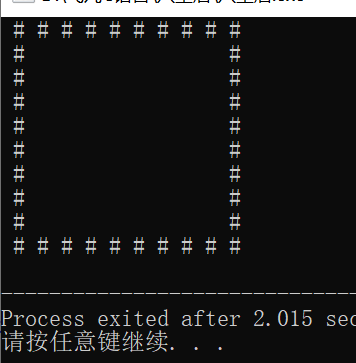2、寻找皇后
typedef struct pos {
int ios;
int jos;
}pos_t;

pos_t pos = {{-1, -1}, {-1, 0}, {-1, 1} }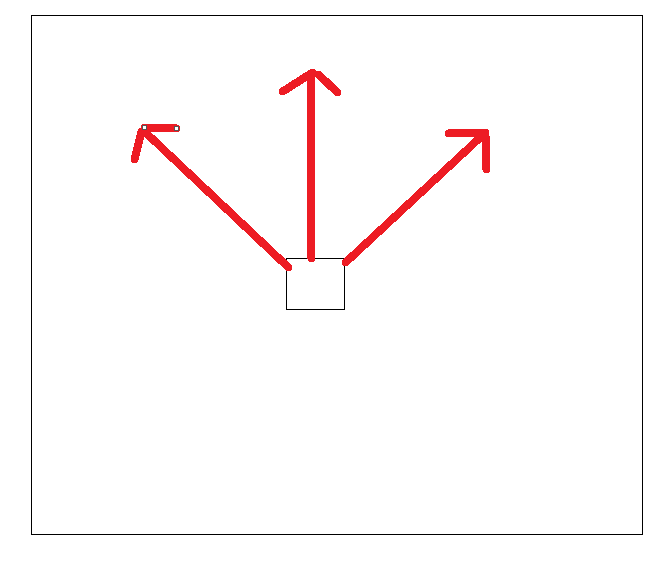在这三个方向上寻找皇后，若发现皇后，则清空上一行皇后的位置 ，重新定义位置。这样递归执行。
int check(int i, int j) {
int ret = 1;//ret为1时：未找到皇后
int p;//寻找的方向

for (p=0; ret && p<3; p++) {
int newi = i;
int newj = j;
while (ret && board[newi][newj]!='#') {
newi += pos[p].ios;
newj += pos[p].jos;
if(board[newi][newj] == '@')//@为皇后
ret = 0;//ret为0：找到皇后
}
}
return ret;
}

void find(int i) {
int j;
if (i == 9) {
show();
getchar();/*getchar()函数等待输入直到按回车才结束，
回车前的所有输入字符都会逐个显示在屏幕上*/
}else
for (j=1; j<=N; j++) {
if( check(i, j) ) {
board[i][j] = '@';
find(i+1);
board[i][j] = ' ';
}
}
}

3、完整代码
#include <stdio.h>
#include <stdlib.h>

#define N 8
char board[N+2][N+2];//定义全局变量

typedef struct pos {
int ios;
int jos;
}pos_t;

pos_t pos = { {-1, -1}, {-1, 0}, {-1, 1} };

//制作棋盘 初始化二维数组
void init() {
int i, j;
for(i=0; i<N+2; i++) {
board[i] = '#';
board[N+1][i] = '#';
board[i] = '#';
board[i][N+1] = '#';
}
for(i=1; i<=N; i++) {
for(j=1; j<=N; j++)
board[i][j] = ' ';
}
}

void show() {
int i, j;
static int cnt = 0;
for (i=0; i<N+2; i++) {
for (j=0; j<N+2; j++)
printf(" %c", board[i][j]);
printf("\n");
}
printf("cnt = %d\n", ++cnt);
}

int check(int i, int j) {
int ret = 1;//ret为1时：未找到皇后
int p;//寻找的方向

for (p=0; ret && p<3; p++) {
int newi = i;
int newj = j;
while (ret && board[newi][newj]!='#') {
newi += pos[p].ios;
newj += pos[p].jos;
if(board[newi][newj] == '@')//@为皇后
ret = 0;//ret为0：找到皇后
}
}
return ret;
}

void find(int i) {
int j;
if (i == 9) {
show();
getchar();
}else
for (j=1; j<=N; j++) {
if( check(i, j) ) {
board[i][j] = '@';
find(i+1);
board[i][j] = ' ';
}
}
}

int main(void){
init();
find(1);
return 0;
}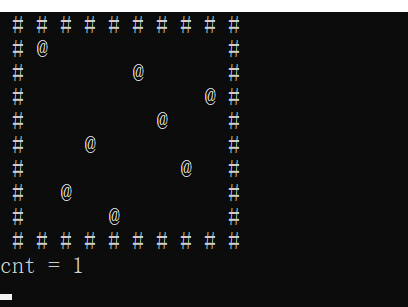按回车，看每一种的结果
直到最后一种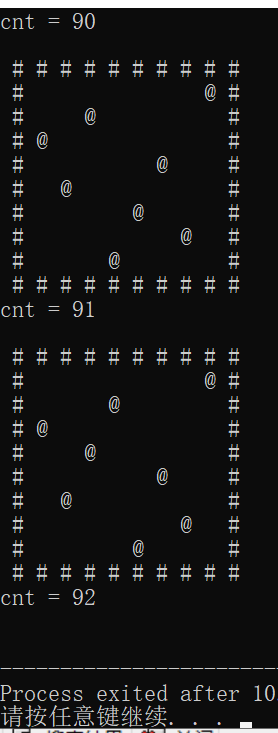共92种摆法


展开全文递归
• #include #include int queenPlaces; //存放92种皇后棋子的...当ithQueen=2，i=2这段代码中在putQueen函数里的第二个皇后放置时放置在棋盘的第二列第三行会使第五个皇后无法放置那具体是根据那条语句回溯？c
• 算法分析：数组a、b、c分别用来标记冲突，a数组代表列冲突，从a~a代表第0列到第7列，如果某列上已经有皇后，则为1，否则为0； 　数组b代表主对角线冲突，为b[i-j+7]（行号-列号+7），主对角线共有15条，即从b...
• 这个是递归思路，你也可以尝试用试验与回溯法做说明下，用一维数组表示棋盘的行，数组的值表示列#includeint is_safe(int *q,int row,int col)/*这个函数是判断各个皇后间是否安全*/{int rr;for(rr=0;rr{if(col==q...
• 99种可能全显示的c语言八皇后问题，数据结构作业
• 八皇后问题是在8*8的国际象棋的棋盘上放置8个皇后，有多少种不同的放置方法，要求它们互相都不冲突（冲突是指在某一行或者某一列或者某一条斜线上出现两个皇后，因为这两个皇后可以互相吃掉对方）。其中行号和列号都...二维数组
• 这是我自己写的八皇后，可以输出全部的解，使用c语言编的，使用回缩算法
• 用短小精悍的C语言代码实现世界著名的八皇后问题
• c语言解决八皇后问题 有代码 有解释 适合初学者用
• 这是用c语言写的八皇后代码，里面增加了计时功能，可以直接运行使用...

# c语言八皇后问题c语言 订阅